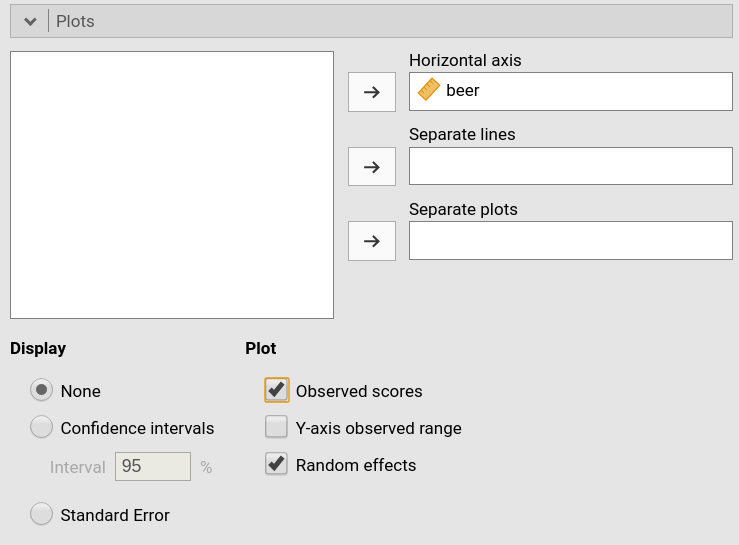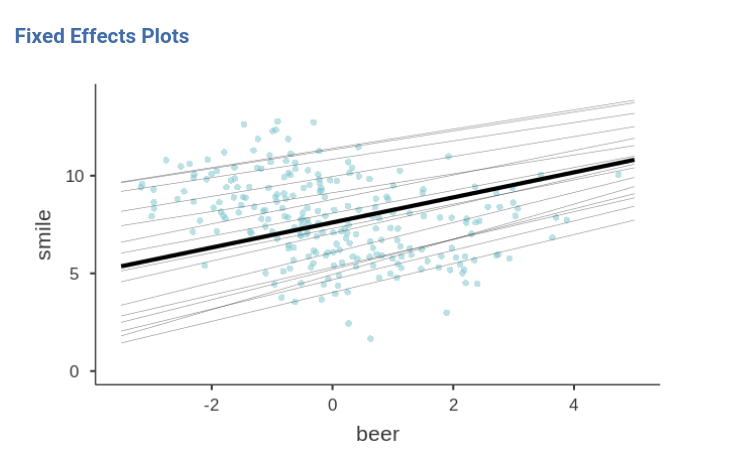keywords Mixed models, hierarchical linear model, multilevel model, simple slopes

GALMj version ≥ 0.9.7

In this example we work out the analysis of some clustered data estimating a mixed model (also called hierarchical linear model or multilevel model) on some simulated (silly) data. We use the GAMLj module in Jamovi. One can follow the example by downloading the file beers at bars and open it in jamovi. Be sure to install GAMLj module from within jamovi library.

Data can also be opened within jamovi in the jamovi data library, with the name Beers.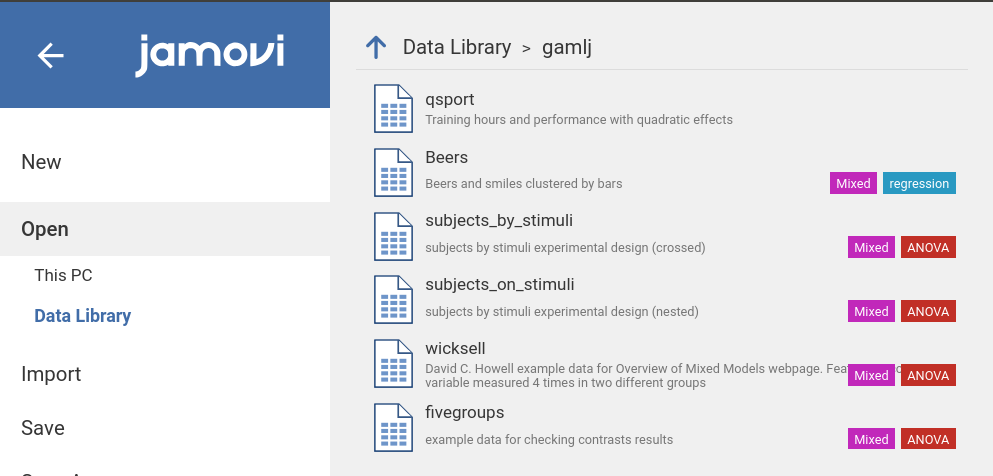# The research design

Imagine we sampled a number of bars (15 in this example) in a city, and in each bar we measured how many beers customers consumed that evening and how many smiles they were producing for a give time unit (say every minute). The aim of the analysis is to estimate the relationship between number of beers and number of smiles, expecting a positive relationship.

We have then 15 bars, each including a different number of customers In the data set, the classification of customers in bars is contained in the variable bar. The frequencies of customers in each bar is in the next table (in jamovi descriptives, tick frequencies table) .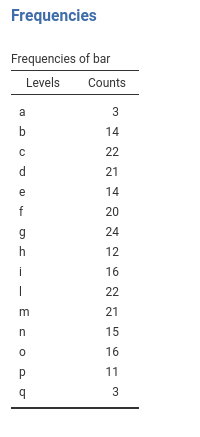Number of beers and number of smiles are recorded in the dataset as beer and smile, respectively, with the following descriptives and distributions.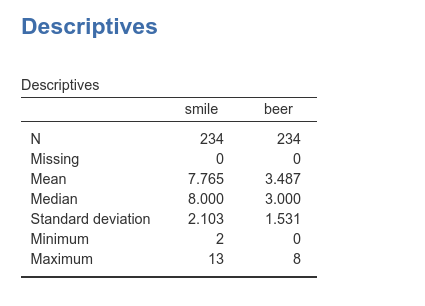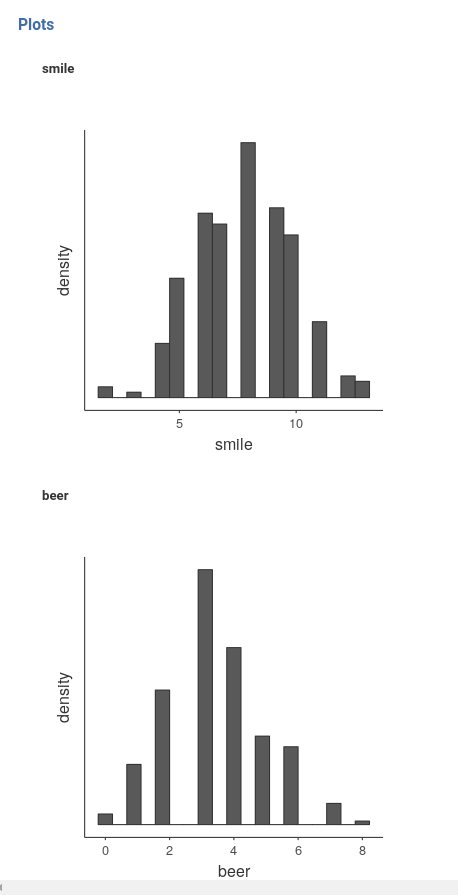# Understanding the problem

If we ignore for a moment the fact that we sampled customers within bars, the analytic problem boils down to a simple regression, with smile as dependent variable and beer as independent variable.

By looking at the scatterplot (in jamovi Exploration -> scatr::Scatterplot)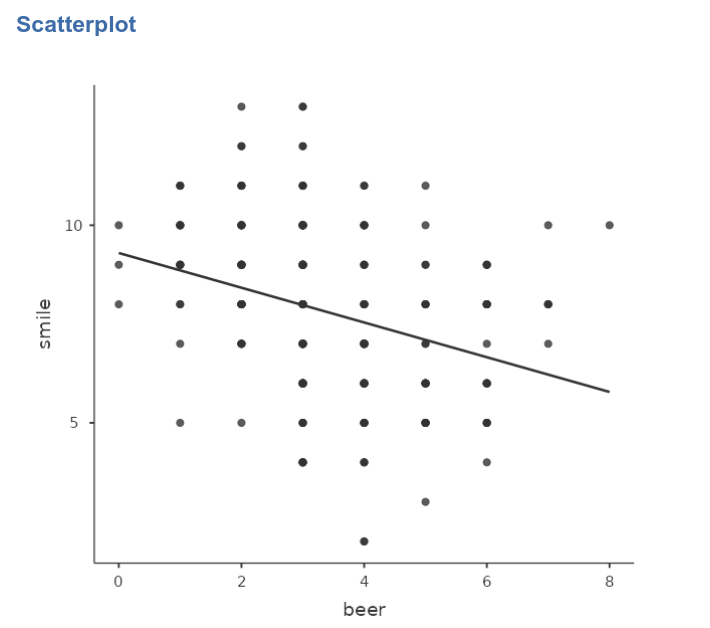we can see that there seems to be a negative relationship between the two variables. A Simple regression ((in jamovi Linear Models -> GAMLj::General Linear Model)) confirms this impression.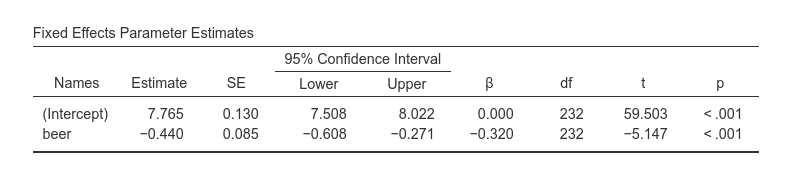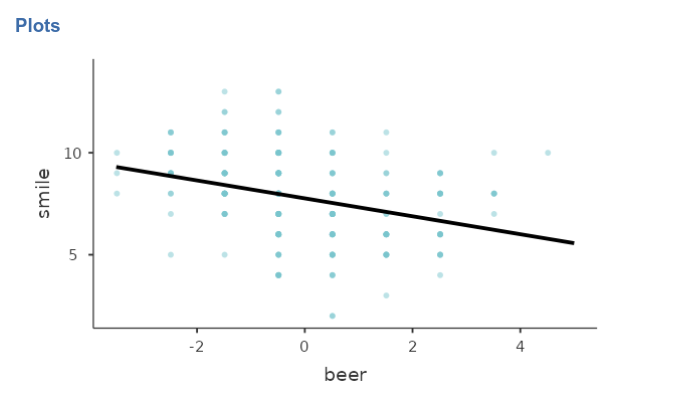The problem with this analysis is that it does not consider the clustering of the data, that is, that customers are grouped within bars. If customers within a bar are more similar in their scores than customers across bars, data show dependency and thus the GLM we ran would be biased. We have to take clustering into the account.

To get the feeling of possible effects of clustering, let’s first look at the distributions of smile and beer within each bar (in jamovi Exploration -> Descriptives, put bar in split by field).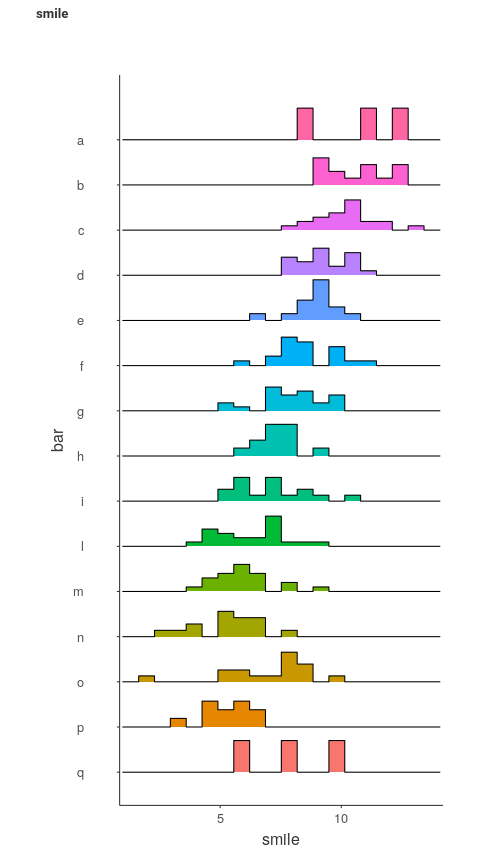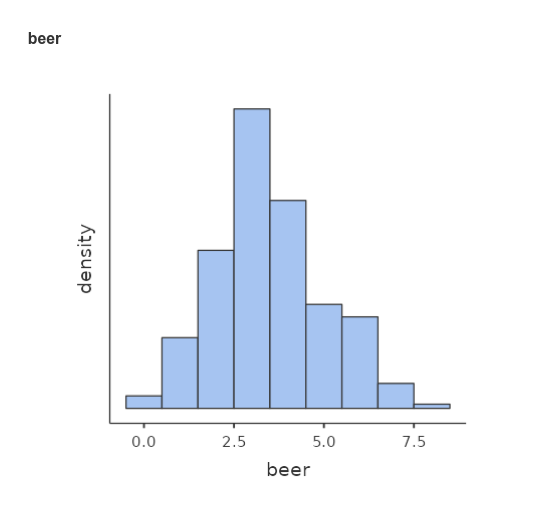We can notice that bars tend to have different means both in the smile and in the beer variables, pointing to possible dependency in the data.

Furthermore, a scatterplot highlighting the bars classification seems to suggest that the points representing scores (# of smiles given the # of beers) are clustered within bars, and also reveals that a model in which each bar is allowed to express a different regression line would fit the data much better than a model with only one regression line, fixed for every bar.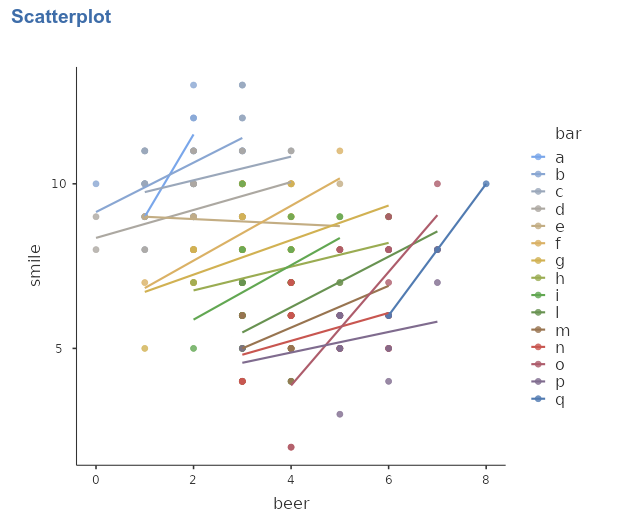# Mixed model

The mixed model allows to obtain exactly what we need here: estimating the relationship between beers and smiles by fitting a regression line within each bar, and then averaging the regression lines to obtain an overall effect of beer on smile. The mixed model accomplishes that by letting the regression coefficients to vary from cluster to cluster, thus estimating different lines for different bars.

The coefficients that vary from cluster to cluster are defined as random coefficients, and their mean (fixed expected value) are defined as fixed coefficients.

Because a simple regression line has two coefficients (the intercept and the slope) we can let the intercept (or constant term) to vary across clusters, the slope, or both. Practically, we define the intercept, or the slope (of beer), or both as random coefficients.

Because we are interested in the overall effect of beer on smile, we want the effect of beer to be also a fixed effect, that is a average slope estimated for across bars. If the beer slope is allowed to vary from bar to bar (i.e. it is set to be random), then the fixed effect should be interpreted as the average slope, averaged across clusters. If the beer slope is not random, then the fixed effect is simply the beer effect estimated across participants.

# Random Intercepts Model

## Set up

We start simply by allowing only the intercepts to vary. This model captures the situation depicted in the scatter-plot by bars.We can see that the regression lines plotted in the graph have different intercepts but they are all perfectly parallel, meaning that they have the same (fixed) slope. This model is called __random intercepts_ model to signal that only the intercepts are allowed to vary from cluster to cluster.

In order to estimate the model with jamovi, we first need to set each variable in the right field.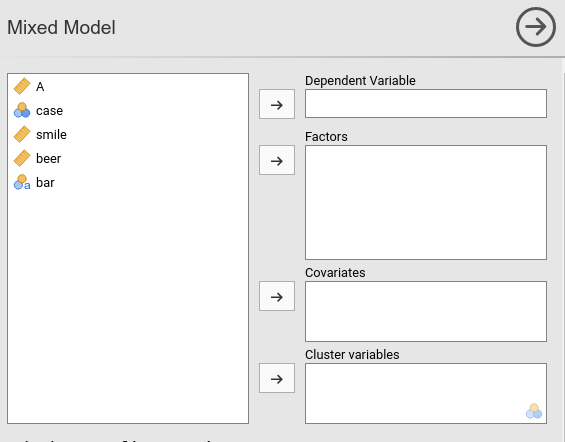First we put smile in the Dependent Variable field and beer in the Covariates field. When a variable is put in the Covariates field, it is treated as a continuous quantitative variable (as.numeric() in R). Had we had a categorical independent variable, we would have put it in “Factors”, so that proper coding of the groups would be obtained (as.factor() in R).

After that, we define bar as the clustering (grouping) variable, by putting it in the Cluster field.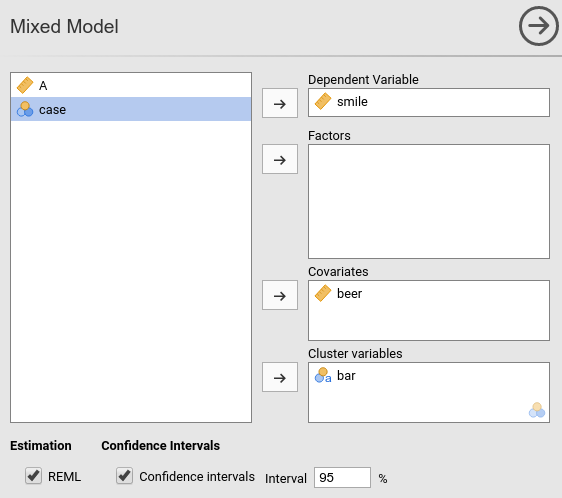If we now look at the results panel, we see that the model definition is not completed yet.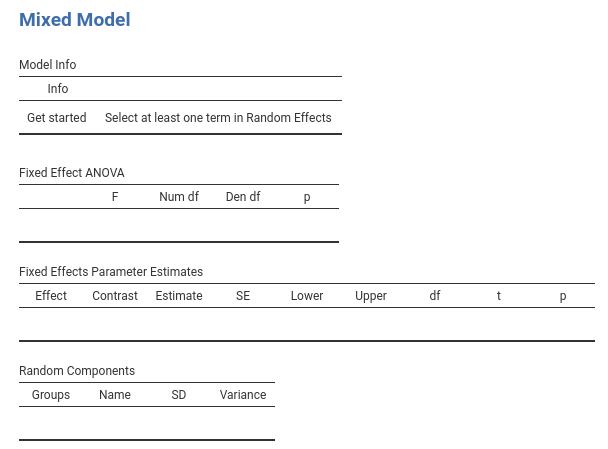We need to specify the random component, that is we should set which coefficient are random. We do that by expanding the Random Effects tab.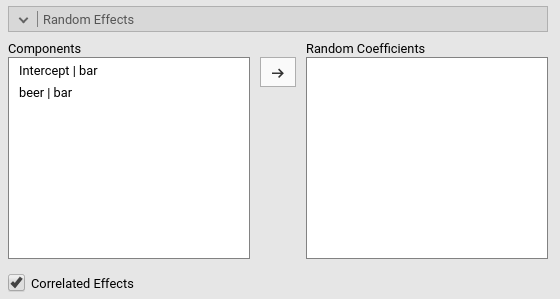On the left side, under Components we find all possible random effects allowed in the model already prepared by jamovi. In our example, they are the intercept random across bars, and the slope of beer random across bars. Jamovi uses the R formulation of random effects as implemented by the lme4 R package. The bar | means random across, thus we can read the “components” as Intercept random across bar, and beer slope random across bar.

Because we decided to start with a random intercept model, we just select the first line in components and push it to the Random Coefficients field.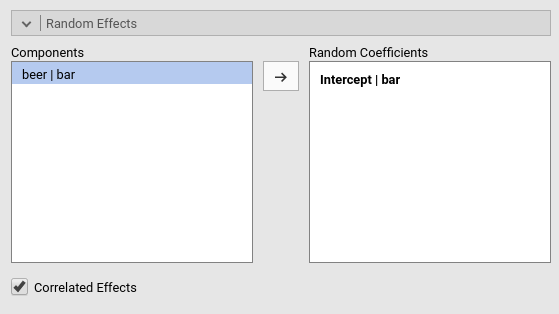At this point, the model is estimated and the results appear in the results panel. Before inspecting the results, we have a look at the fixed effects definition, by expanding the Fixed Effects’ tab.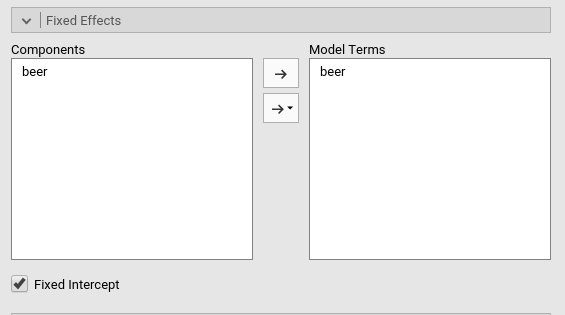Although we did not do anything about the fixed effects, jamovi automatically includes all independent variables defined in Covariates or in Factor in the fixed effects model. Obviously, when the models are complex, one can tweak the model terms to suit the analysis aims.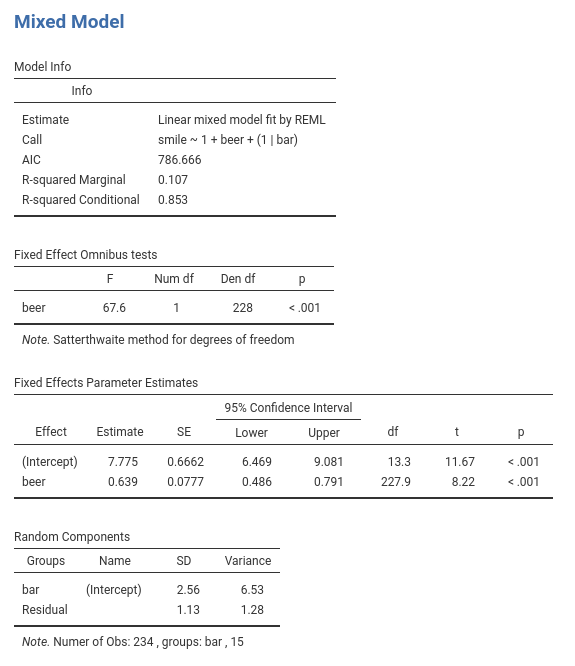## Results

The first table in the output contains info about the model and the estimation.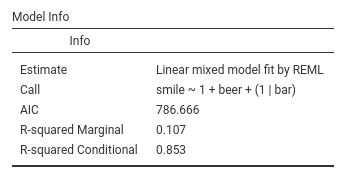• The Call row displays the model in lme4 R package formulation. This can be useful to re-run the same analysis in R (not using GAMLj module).
• The AIC row displays the Aikeke Information Criterion, which can be useful to evaluate the model, especially in comparison with other models. Details can be found in GAMLj docs and in [Zuur et. al , 2009] al.](http://www.springer.com/la/book/9780387874579)
• R-marginal and R-conditional are proportion of reduced error, or pseudo-$$R^2$$. They are described in Johnson (2014) and implemented in piecewiseSEM. For our purposes, we can interpret them as follows: R-marginal is the variance explained by the fixed effects over the total (expected) variance of the dependent variable. The R-conditional is the variance explained by the fixed and the random effects together over the total (expected) variance of the dependent variable. In our example, the fixed effects do not explain much (.107), but the overall model (fixed+random) captures a fairly big share of the variance (.853).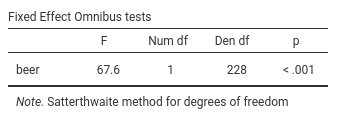Fixed effects ANOVA gives the F-tests associated with the model fixed effects. Here we see that beer has a statistical significant effect (on average) on number of smiles.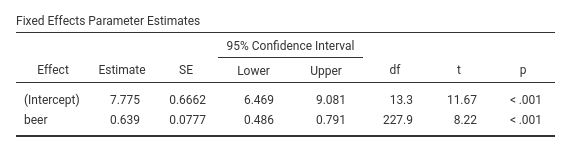As regards the degrees of freedom (nobody cares about them, I know), jamovi mixed model tries to use Satterthwaite approximation as much as possible, but for complex models it may fail. When that happens, Kenword-Roger approximation is used and, if the latter does not fail, F-tests are computed. A note signals which approximation is used.

Fixed effects Parameters Estimates gives the fixed B coefficients, the fixed (average) intercept, t-tests associated with the model fixed effects. Accordingly, we can say that averaging across bars, beer has a statistical significant effect on number of smiles, such that for each beer more, people smiles 0.548 smiles more.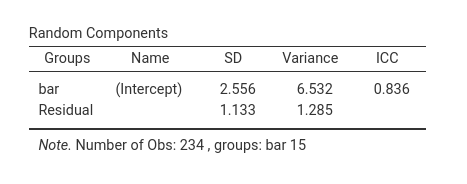As regards the intercept (which people usually ignore) we should interpret it as the expected number of smiles for the average number of beers drunken. This can be surprising because one expects the intercept to be the expected value of Y when X=0. It is, of course, also here but jamovi mixed model module centers the continuous variables by default. Thus, X=0 means X=mean. Indeed, in the Covariates Scaling tab we see that: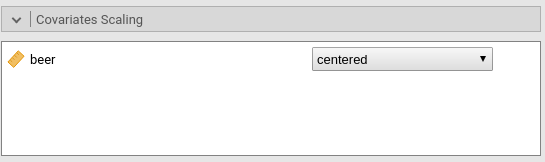Options are available to scale the covariates, by centering it or standardizing it. The options "cluster-based-*" operate the re-scaling (centering or standardizing) within each cluster rather than on the sample as a whole.

The Random Component display the variances and SD of the random coefficients, in this case of the random intercepts. From the table we can see that there is a good variance of the intercepts ($${\sigma_a}^2$$=6.53), thus we did well in letting the intercepts vary from cluster to cluster. ($${\sigma_a}^2$$=6.53) can be reported as an intra-class correlation by dividing it by the sum of itself and the residual variance ($$\sigma^2$$), that is $$v_{ic}={{\sigma_a}^2 \over {{\sigma_a}^2+{\sigma}^2}}$$

# Random Slopes Model

## Set up

We can now expand the model by letting the slopes to vary as well. We just need to update the definition of the random coefficients in the Random Effects tab, adding also the beer|bar term.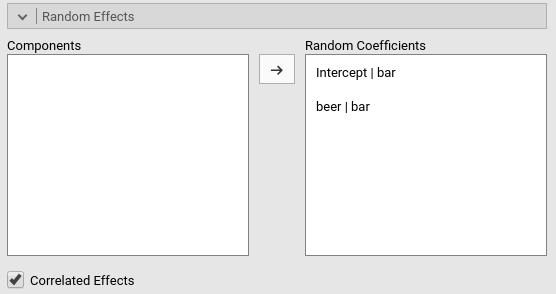Notice that we have now two random effects, that can be correlated or fixed to be uncorrelated (i.e. variance components model). The option Correlated Effects set up the correlation of the random effects. In this version of GAMLj module, the random coefficients can be either all correlated or all not correlated. Future versions will allow more freedom in the definition of the random covariance structure. People experienced in SPSS Mixed would recognize these two options to be UN and VC` in SPSS syntax, respectively.

## Results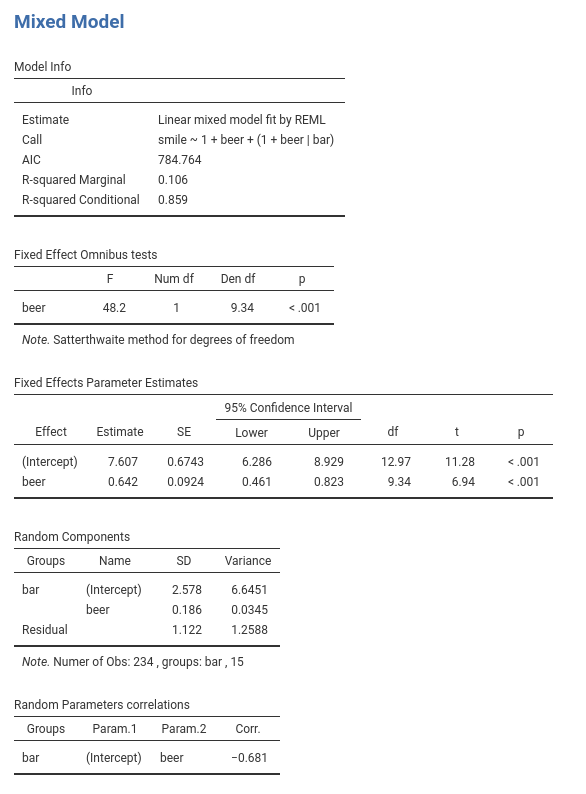Results are substantially the same, showing that the variability of the slopes do no influence the interpretation of the results in a substantial way. We can notice, however, that the DF of the tests are different as compared with the random intercepts model. This is due to the fact that now the fixed slope 0.584 is computed as the average of the random slopes, and thus its inferential sample is much smaller.

In the ‘Random Components’ table we see a small variance of beer $${\sigma_b}^2$$=0.0345, indicating that slopes do not vary much. Nonetheless, their variability it is not null, so allowing them to be random increases our model fit. This is also testified by a (slightly) smaller AIC (from 786 to 784) and a larger $$R^2$$-conditional (from .81 to .82). Because we do not test any inferential hypothesis on these variances, it is better to keep them in the model. As they say: if it ain’t broken, don’t fix it.

Finally, a correlation between intercepts and slopes can be observed, $$r$$=-.681, indicating that bars where people smile more on average (intercept) are the bars were the effect of beer is smaller.

The final model, with random intercepts and slopes, would capture the data with several slopes, with very different intercepts and slightly variable slopes.